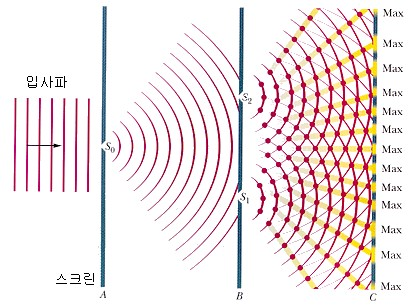# Young’s Double Slit

You can adjust the spacing and position of the slits.

## Young’s double slit experiment

Young’s interference experiment is as follows: The light diffracted from the small holes S0 on-screen ‘A’ passes through the small holes S1 and S2.
Light passing through the two small holes overlaps between screens B and C, creating an interference fringe on screen C.## Constructive interference

When the waves arriving from the two slits are in the same phase, a bright pattern is formed.
To cause constructive interference, the path difference d·sin θ must be ‘0’ or an integer multiple of the wavelength.

Constructive interference $$d\cdot sin(\theta )\,=\,n\lambda$$ (n = 0, 1, 2…)

position $${y }_{ n }\,=\,\frac {n\lambda D }{ d}$$ (n = 0, 1, 2…)

d: Slit spacing (m)
λ: wavelength of light (m)
D: Distance from slit to screen (m)

When ‘n = 0’, the diffraction angle of the central axis ‘θ = 0’.

## Destructive interference

When the waves arriving from the two slits are in the opposite phase, a dark pattern is formed
To cause destructive interference, the difference in the path between the two sources must be an odd multiple of the half wavelength.

Destructive interference $$d\cdot sin(\theta )\,=\,(n+\frac {1 }{ 2} )\lambda$$ (n = 0, 1, 2…)

position $${y }_{ n }\, =\, \frac {\left( n+\frac {1 }{ 2} \right) \lambda D }{ d}$$ (n = 0 , 1, 2…)

d: Slit spacing (m)